CAT  >  Inequalities - Important Formulae of Number system - Free

# Inequalities - Important Formulae of Number system - Free Notes | Study Quantitative Aptitude (Quant) - CAT

## Document Description: Inequalities - Important Formulae of Number system - Free for CAT 2022 is part of 2.6 Inequalities for Quantitative Aptitude (Quant) preparation. The notes and questions for Inequalities - Important Formulae of Number system - Free have been prepared according to the CAT exam syllabus. Information about Inequalities - Important Formulae of Number system - Free covers topics like and Inequalities - Important Formulae of Number system - Free Example, for CAT 2022 Exam. Find important definitions, questions, notes, meanings, examples, exercises and tests below for Inequalities - Important Formulae of Number system - Free.

Introduction of Inequalities - Important Formulae of Number system - Free in English is available as part of our Quantitative Aptitude (Quant) for CAT & Inequalities - Important Formulae of Number system - Free in Hindi for Quantitative Aptitude (Quant) course. Download more important topics related with 2.6 Inequalities, notes, lectures and mock test series for CAT Exam by signing up for free. CAT: Inequalities - Important Formulae of Number system - Free Notes | Study Quantitative Aptitude (Quant) - CAT
 1 Crore+ students have signed up on EduRev. Have you?

If a > b and c > 0,
⇒ a + c > b + c
⇒ a - c > b - c
⇒ ac > bc
⇒ a/c > b/c
If a, b ≥ 0, then an > bn and 1/an < 1/bn, where n is positive.
a < b and x > 0, then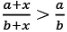a > b and x > 0, then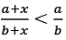Modular Inequalities
|x- y| = |y - x|
|x. y| = |x|  . |y|
|x+ y| < |x| + |y|
|x+ y| > |x| - |y|

(x – a) (x – b) > 0 {a < b}
⇒ (x < a) U (x > b)
(x – a) (x – b) < 0 {a > b}
⇒ a < x < b
For any set of positive numbers: AM≥GM≥HM
⇒ (a1+a2+ ….+an)/n ≥(a1.a2. …..an)1/n
If a and b are positive quantities, then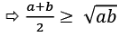If a,b,c,d are positive quantities, then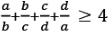⇒ a4 + b4 + c4 + d4 ≥ 4abcd
If a,b,c …. k are n positive quantities and m is a natural number, then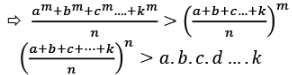EduRev's Tip:

•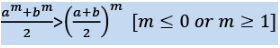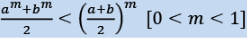• For any positive integer n, 2 ≤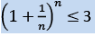• ambncp……..will be greatest when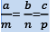• If a > b and both are natural numbers, then
⇒ ab < ba {Except 32 > 23 & 42 = 24}
• (n!)2 ≥ nn
•  If the sum of two or more positive quantities is constant, their product is greatest when they are equal and if their product is constant then their sum is the least when the numbers are equal.
⇒ If x + y = k, then xy is greatest when x = y
⇒ If xy = k, then x + y is least when x = y
The document Inequalities - Important Formulae of Number system - Free Notes | Study Quantitative Aptitude (Quant) - CAT is a part of the CAT Course Quantitative Aptitude (Quant).
All you need of CAT at this link: CAT

## Quantitative Aptitude (Quant)

162 videos|152 docs|131 tests
 Use Code STAYHOME200 and get INR 200 additional OFF

## Quantitative Aptitude (Quant)

162 videos|152 docs|131 tests

### How to Prepare for CAT

Read our guide to prepare for CAT which is created by Toppers & the best Teachers

Track your progress, build streaks, highlight & save important lessons and more!

,

,

,

,

,

,

,

,

,

,

,

,

,

,

,

,

,

,

,

,

,

;